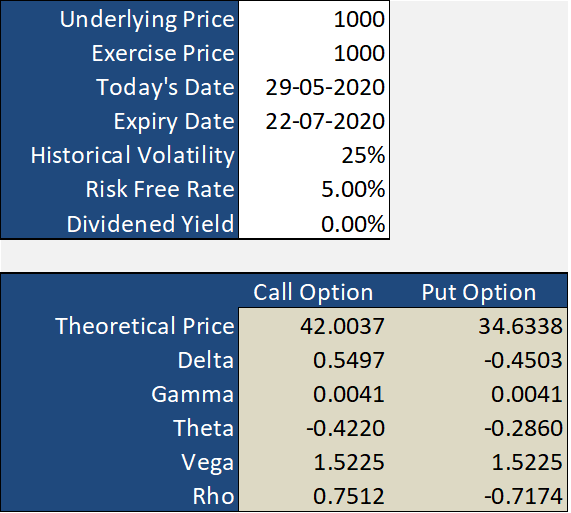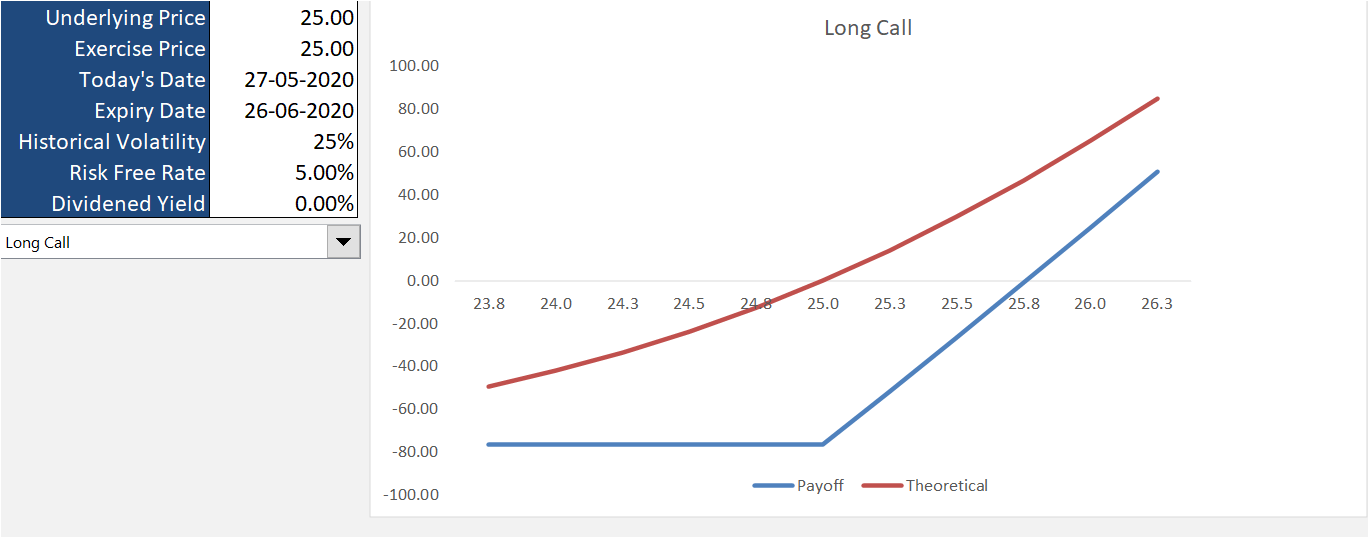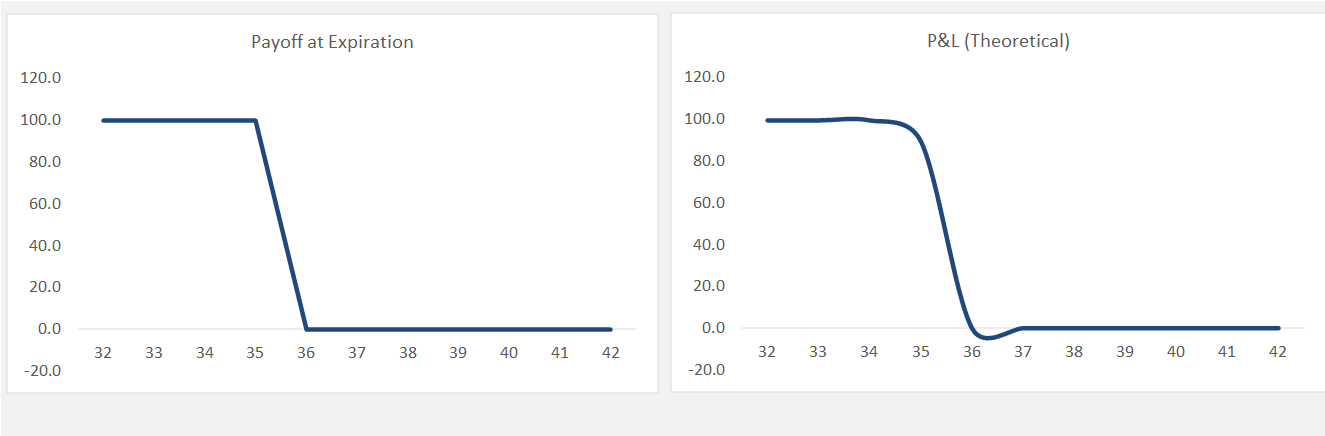# Options Premium Calculator using Black Scholes Model: Excel Sheet

Options belong to the derivative family of financial instruments and are considered relatively ‘complex’ when compared to stocks and commodities. The complexity arises due to the unique option pricing methodology which depends on several factors. In this post, we’ll explore the Options premium calculator spreadsheet that greatly simplifies options pricing as well as payoff calculation. The spreadsheet is based on Black Scholes Model and can be downloaded from the end of this post.## What is Black Scholes Model?

The Black Scholes model is a mathematical model to determine the theoretical price of the call and put options. The pricing is calculated based on the below 6 factors:

1. Underlying Price
2. Strike price
3. Time to Expiration (in years)
4. Risk-Free Interest Rate
5. Dividend Yield
6. Volatility

There are two primary models used to estimate the pricing of options – Binomial model and Black Scholes model. Out of the two, the Black Scholes model is more prevalent. The popularity of the Black Scholes model can be estimated from the fact that the developer of this model; Fischer Black, Myron Scholes, and Robert Merton won the Nobel Prize in Economics for their work.

The mathematics behind the Black Scholes model is quite complex and there isn’t any practical need to know every sheer detail behind it. Moreover, the spreadsheet we are going to discuss in the next section simplifies this pricing model (using several background calculations), so that you can readily use it.

Also Read: Options strategy payoff calculator Excel Sheet

Let’s understand this powerful spreadsheet and the functionalities it offers. I would not go into every minute detail regarding how things are calculated, rather I would stick to usage of this sheet.

In case, you want to explore the calculations, the VBA code is unlocked.

The Options premium calculator spreadsheet has three primary workbooks. Let’s understand them one by one:

### Basic Workbook

The basic workbook calculates the theoretical call and put options price and greeks based on the input you provide (Cells C3 TO C9).For accurate calculations, make sure you enter correct values in the white cells (C3 to C9).

In addition to the prices and greeks, you can also calculate implied volatility (IV) of call and put options in this sheet. In order to do that, enter the current market price of the option strikes in cells C20 and D20 (white cells) and see the result in the row below it.### PayoffGraphs Workbook

To use this sheet, you’ll need to enter the same variables you entered in the ‘basic workbook’, along with the type of option position from the dropdown.### Strategies Workbook

The strategies workbook allows you to combine various option legs and calculate the effective payoff. You can add up to 10 option positions. Not only it calculates the payoff, but also theoretical prices, greeks, and volatility.

Similar to other sheets, you need to enter the inputs in the white cells.